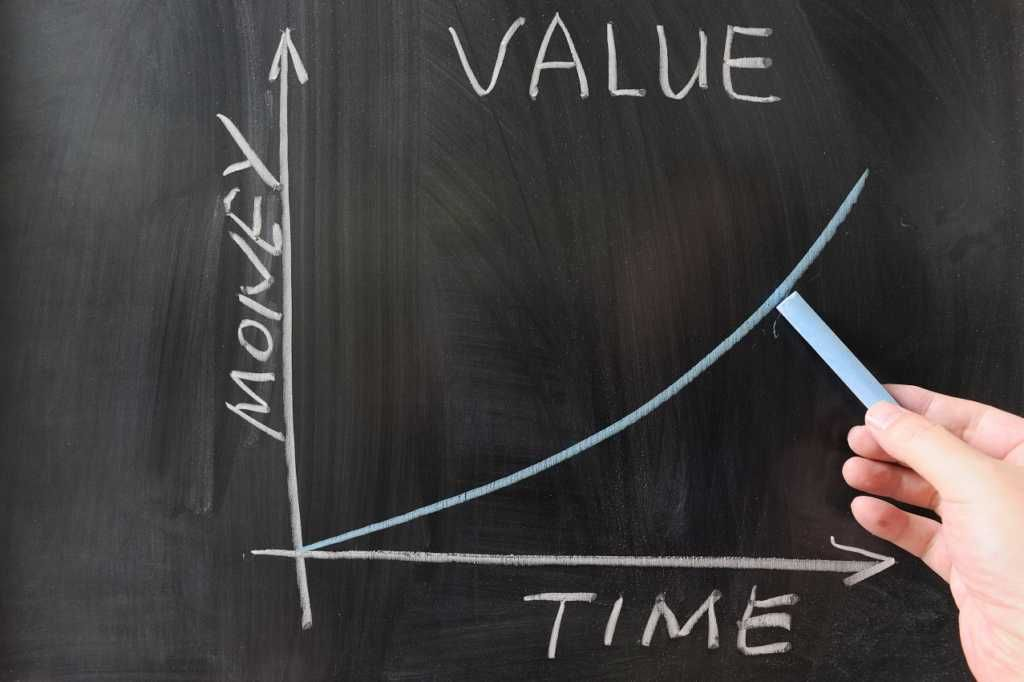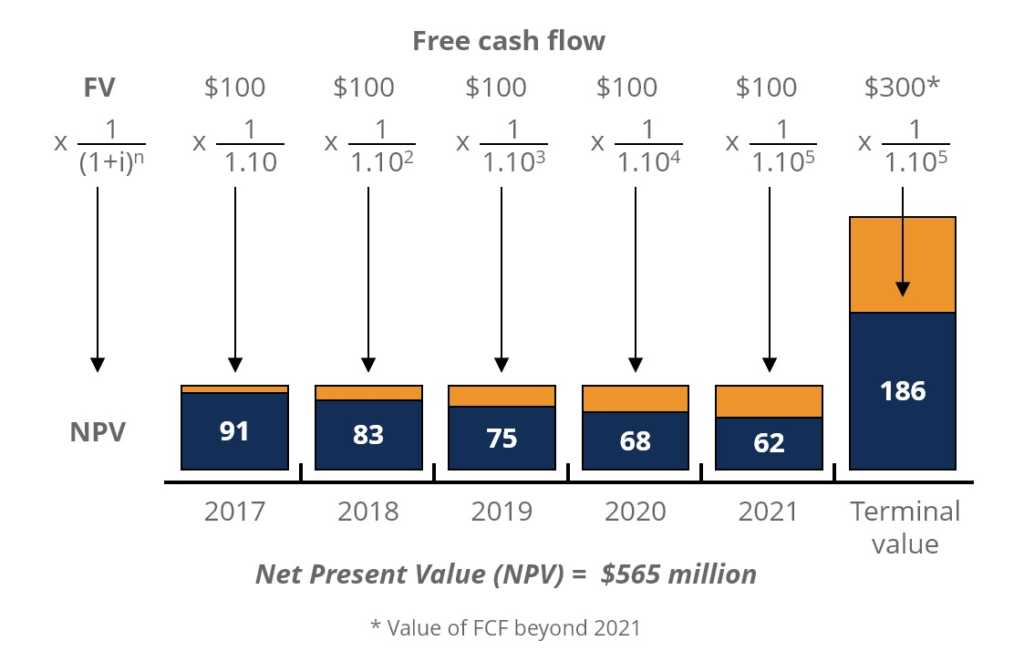# Time Value of Money

Money in the present is worth more than the same sum of money to be received in the future

## What is the Time Value of Money?

The time value of money is a basic financial concept that holds that money in the present is worth more than the same sum of money to be received in the future. This is true because money that you have right now can be invested and earn a return, thus creating a larger amount of money in the future. (Also, with future money, there is the additional risk that the money may never actually be received, for one reason or another.) The time value of money is sometimes referred to as the net present value (NPV) of money.### How the Time Value of Money Works

A simple example can be used to show the time value of money. Assume that someone offers to pay you one of two ways for some work you are doing for them: They will either pay you \$1,000 now or \$1,100 one year from now.

Which pay option should you take? It depends on what kind of investment return you can earn on the money at the present time. Since \$1,100 is 110% of \$1,000, then if you believe you can make more than a 10% return on the money by investing it over the next year, you should opt to take the \$1,000 now. On the other hand, if you don’t think you could earn more than 9% in the next year by investing the money, then you should take the future payment of \$1,100 – as long as you trust the person to pay you then.

### Time Value and Purchasing Power

The time value of money is also related to the concepts of inflation and purchasing power. Both factors need to be taken into consideration along with whatever rate of return may be realized by investing the money.

Why is this important? Because inflation constantly erodes the value, and therefore the purchasing power, of money. It is best exemplified by the prices of commodities such as gas or food. If, for example, you were given a certificate for \$100 of free gasoline in 1990, you could have bought a lot more gallons of gas than you could have if you were given \$100 of free gas a decade later.Inflation and purchasing power must be factored in when you invest money because to calculate your real return on an investment, you must subtract the rate of inflation from whatever percentage return you earn on your money. If the rate of inflation is actually higher than the rate of your investment return, then even though your investment shows a nominal positive return, you are actually losing money in terms of purchasing power. For example, if you earn a 10% on investments, but the rate of inflation is 15%, you’re actually losing 5% in purchasing power each year (10% – 15% = -5%).

### Time Value of Money Formula

The time value of money is an important concept not just for individuals, but also for making business decisions. Companies consider the time value of money in making decisions about investing in new product development, acquiring new business equipment or facilities, and establishing credit terms for the sale of their products or services.

A specific formula can be used for calculating the future value of money so that it can be compared to the present value:Where:

FV = the future value of money
PV = the present value
i = the interest rate or other return that can be earned on the money
t = the number of years to take into consideration
n = the number of compounding periods of interest per year

Using the formula above, let’s look at an example where you have \$5,000 and can expect to earn 5% interest on that sum each year for the next two years. Assuming the interest is only compounded annually, the future value of your \$5,000 today can be calculated as follows:

FV = \$5,000 x (1 + (5% / 1) ^ (1 x 2) = \$5,512.50

### Present Value of Future Money Formula

The formula can also be used to calculate the present value of money to be received in the future. You simply divide the future value rather than multiplying the present value. This can be helpful in considering two varying present and future amounts. In our original example, we considered the options of someone paying your \$1,000 today versus \$1,100 a year from now. If you could earn 5% on investing the money now, and wanted to know what present value would equal the future value of \$1,100 – or how much money you would need in hand now in order to have \$1,100 a year from now – the formula would be as follows:

PV = \$1,100 / (1 + (5% / 1) ^ (1 x 1) = \$1,047

The calculation above shows you that, with an available return of 5% annually, you would need to receive \$1,047 in the present to equal the future value of \$1,100 to be received a year from now.

To make things easy for you, there are a number of online calculators to figure the future value or present value of money.

### Net Present Value Example

Below is an illustration of what the Net Present Value of a series of cash flows looks like. As you can see, the Future Value of cash flows are listed across the top of the diagram and the Present Value of cash flows are shown in blue bars along the bottom of the diagram.This example is taken from CFI’s Free Introduction to Corporate Finance Course, which covers the topic in more detail.

We hope you’ve enjoyed CFI’s explanation of the time value of money. To learn more about money and investing, check out the following resources:

• Forecasting Methods
• NPV Formula
• Valuation Methods

### Valuation Techniques

Learn the most important valuation techniques in CFI’s Business Valuation course!

Step by step instruction on how the professionals on Wall Street value a company.

Learn valuation the easy way with templates and step by step instruction!